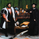Dilihat 5227

There are three popular styles of Pair trading:

The correlation based strategy is to short the outperforming instrument and go long on the underperforming one
whenever the temporary correlation weakens which means one instrument going up and another going down.
Here, instead of two different instruments two timeframes of the same instrument are used, lower and higher.
In order to calculate the trade size, we would need to calculate hedge_ratio,
which is simply the ratio of the closing price of the instrument in the current tf to the higher tf.
So whenever we want to go short on spread we’ll short 1 unit of current tf and go long on hedge ratio times units of higher tf.

In order to generate trading signals, we need to define our trading logic.
So, we’ll go long on the spread when the hedge ratio goes below its p rolling mean by mult times of its
rolling standard deviation and vice versa.
Note that both p and mult can be changed as per your selection of instruments.

NOTE:
There is a minor problem to it in Pinescript. After a while the signal line goes horizontal and the two bounds, upper and lower, converge unless you update the time frame.
Kepp me in the know if you find a solution to this problem)

There are three popular styles of Pair trading:

The correlation based strategy is to short the outperforming instrument and go long on the underperforming one
whenever the temporary correlation weakens which means one instrument going up and another going down.
Here, instead of two different instruments two timeframes of the same instrument are used, lower and higher.
In order to calculate the trade size, we would need to calculate hedge_ratio,
which is simply the ratio of the closing price of the instrument in the current tf to the higher tf.
So whenever we want to go short on spread we’ll short 1 unit of current tf and go long on hedge ratio times units of higher tf.

In order to generate trading signals, we need to define our trading logic.
So, we’ll go long on the spread when the hedge ratio goes below its p rolling mean by mult times of its
rolling standard deviation and vice versa.
Note that both p and mult, as well as tf, can be changed as per your selection of instruments.
Skrip open-source

Sejalan dengan semangat TradingView, penulis skrip ini telah mempublikasikannya secara open-source, sehingga para trader dapat memahami dan memverifikasinya. Salut untuk sang penulis! Anda dapat menggunakannya secara gratis, tetapi penggunaan kembali kode ini dalam publikasi diatur oleh Tata Tertib. Anda dapat memfavoritkannya untuk dapat menggunakannya didalam sebuah chart.

Inggin menggunakan skrip ini pada chart?

## KomentarReplaced the line
Y = security(tickerid, customReso, X)
with
Y = security(tickerid, customReso, X, barmerge.gaps_off, barmerge.lookahead_on),
so now the indicator updates as required.
Jawab